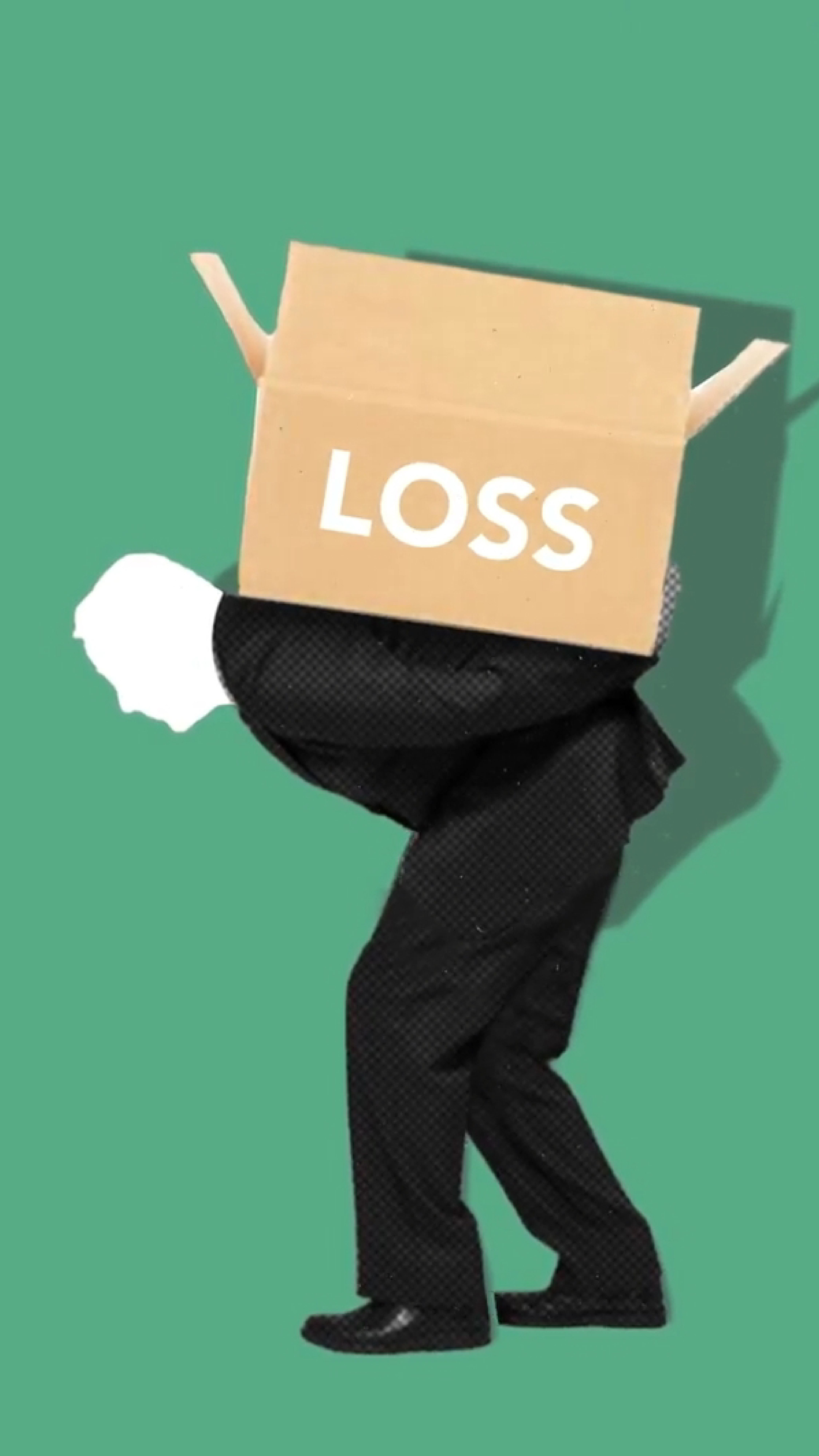# Is microeconomics a lot of math?

Is Microeconomics a Lot of Math? – College Tips and Tricks Blog

# Is Microeconomics a Lot of Math?

Find out the answer to this common question about microeconomics in this detailed article.

## FAQ

What is microeconomics?
Microeconomics is the study of individual economic behavior, such as households and firms, and how they interact with each other in markets.
Is microeconomics a math-intensive subject?
Microeconomics can be, but is not necessarily, math-intensive. Fundamental microeconomic assumptions about scarcity, human choice, rationality, ordinal preferences or exchange do not require any advanced mathematical skills.
What kind of math is involved in microeconomics?
Basic arithmetic, algebra, and graphing are often used in microeconomics to analyze economic concepts such as supply and demand, elasticity, consumer and producer surplus, and profit maximization.
Do I need to be good at math to succeed in microeconomics?
While some math skills are helpful in understanding microeconomics, it is not the only factor in success. Critical thinking, analytical skills, and the ability to apply economic concepts to real-world situations are also important.
Can I still take microeconomics if I’m not good at math?
Yes, you can still take and succeed in microeconomics even if you’re not particularly skilled in math. However, it may require more effort and practice to understand the mathematical concepts involved.

## Math in MicroeconomicsOne of the most common questions among microeconomics students is whether the subject is a lot of math. The answer is not entirely straightforward, as it depends on your academic background, your professor’s teaching style, and your personal learning preferences.

It is true that microeconomics requires some math skills, particularly arithmetic, algebra, and graphing. Students learn how to analyze economic concepts such as supply and demand, elasticity, consumer and producer surplus, and profit maximization using mathematical models and formulas.

However, it is important to note that microeconomics is not all about math. In fact, some of the fundamental assumptions of microeconomics, such as scarcity, human choice, rationality, ordinal preferences, or exchange, do not require any advanced mathematical skills.

Ultimately, your success in microeconomics will depend on your ability to think critically, analyze economic situations, and apply economic concepts to real-world scenarios. Of course, having a solid understanding of math will help, but it is not the only factor in achieving a good grade.

## Mathematical Concepts in MicroeconomicsSome of the most common mathematical concepts students encounter in microeconomics include:

• Supply and demand curves: These are graphical representations of the relationship between the price of a good or service and the quantity that consumers are willing and able to buy and producers are willing and able to sell in a competitive market.
• Elasticity: Elasticity measures the responsiveness of buyers and sellers to changes in market conditions, such as price, income, or availability of substitutes.
• Consumer and producer surplus: Consumer surplus is the difference between what a consumer is willing to pay for a good or service and what they actually pay. Producer surplus is the difference between the market price of a good or service and the minimum price producers are willing to accept.
• Profit maximization: Firms attempt to maximize profits by producing the quantity of output where marginal revenue equals marginal cost.